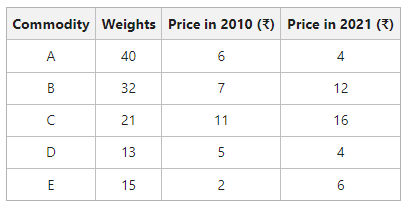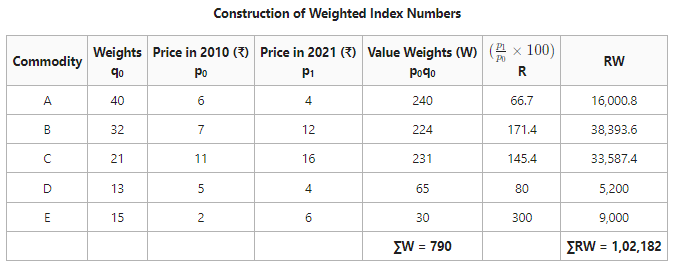# Methods of calculating Weighted Index Numbers

A statistical measure that helps in finding out the percentage change in the values of different variables, such as the price of different goods, production of different goods, etc., over time is known as the Index Number. The percentage change is determined by taking a base year as a reference. This base year is the year of comparison. When an investigator studies different goods simultaneously, then the percentage change is considered the average for all the goods. There are two broad categories of Index Numbers: viz., Simple or Unweighted and Weighted Index Numbers.

According to Spiegel, “An Index Number is a statistical measure designed to show changes in a variable or group of related variables with respect to time, geographic location or other characteristics.”

According to Croxton and Cowden, “Index Numbers are devices for measuring difference in the magnitude of a group of related variables.”

### Weighted Index Numbers

In other index numbers, equal importance is given to each item. However, under Weighted Index Number, rational weights are given to each commodity or item explicitly. These weights indicate the relative importance of the commodities or items included in the determination of the index.

Here, rational weights mean the weights which are perfectly rational for one investigation. However, this weight might be unsuitable for other investigations. In fact, the purpose of the index number and the nature of the data concerned with it helps in deciding the rational weights.

There are two methods through which Weighted Index Numbers can be constructed; viz., Weighted Aggregative Method and Weighted Average of Price Relatives Method.

### 1. Weighted Aggregative Method:

This method involves assigning weights to different items and obtaining weighted aggregate of the prices instead of finding a simple aggregate of prices. Some important methods of constructing Weighted Aggregative Index are as follows:

• Laspeyre’s Method
• Paasche’s Method
• Fisher’s Ideal Method
• Drobish and Bowley’s Method
• Marshall Edgeworth Method
• Walsch’s Method
• Kelly’s Method

Note: According to CBSE Syllabus, we will be only studying Laspeyre’s, Paasche’s, and Fisher’s Methods.

#### i) Laspeyre’s Method

The method of calculating Weighted Index Numbers under which the base year quantities are used as weights of different items is known as Laspeyre’s Method. The formula for Laspeyre’s Price Index is:Here,

P01 = Price Index of the current year

p0 = Price of goods at base year

q0 = Quantity of goods at base year

p1 = Price of goods at the current year

#### ii) Pasche’s Method

The method of calculating Weighted Index Numbers under which the current year’s quantities are used as weights of different items is known as Pasche’s Method. The formula for Pasche’s Price Index is:Here,

P01 = Price Index of the current year

p0 = Price of goods in the base year

q1 = Quantity of goods in the base year

p1 = Price of goods in the current year

#### iii) Fisher’s Method

The method of calculating Weighted Index Numbers under which the combined techniques of Pasche and Laspeyre are used is known as Fisher’s Method. In other words, both the base year and current year’s quantities are used as weights. The formula for Fisher’s Price Index is:Here,

P01 = Price Index of the current year

p0 = Price of goods in the base year

q1 = Quantity of goods in the base year

p1 = Price of goods in the current year

Fisher’s Method is considered the Ideal Method for Constructing Index Numbers.

### 2. Weighted Average of Price Relatives Method:

Under this method, the base year prices of the commodities are taken as the basis to calculate the price relatives for the current year. The calculated price relatives are then multiplied by their respective weights of the items. After that, the products determined are added up and divided by the sum of weights.

The steps required to construct index number through this method are as follows:

1. Firstly, calculate the price relatives of the current year; i.e., divide the price of each commodity in the current year by the price in the base year, and denote the value calculated as R.
2. Now, multiply the price of commodities in the base year (p0) with their respective weights (q0), and denote the value weights by W.
3. After that, multiply the price relatives (R) with value weights (W) and obtain their total; i.e., ∑RW.
4. Determine the total of value weights; i.e., ∑W.
5. Use the following formula to determine Index Number:#### Example:

Use Weighted Relatives Method and determine the index number from the following data for the year 2021 with 2010 as the base year.#### Solution:Weighted Average of Price RelativesThe Index Number of 129.34 shows that there is an increase of 29.34% in the prices in the year 2021 as compared to the year 2010.